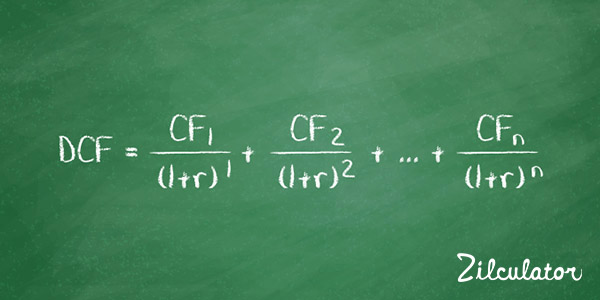# How to Calculate Discounted Cash Flow (DCF)

## Formula & DefinitionDiscounted Cash Flow is a term used to describe what your future cash flow is worth in today's value. This is also known as the present value (PV) of a future cash flow.

Basically, a discounted cash flow is the amount of future cash flow, minus the projected opportunity cost. Your cash flow is always more valuable to you in the present because you can invest it and increase the amount you have.

Calculating a discounted cash flow is a little like calculating your savings account's compound interest. Let's say you put money into a savings account and leave it alone for a year to let it earn interest. After that year is up, you have your original money (your principle) plus the interest that money earned throughout the year. At the end of the second year, your principle and your interest combine to earn compounded interest.

By calculating your discounted cash flow, you can make an educated guess at what the value of your cash flow in a particular time in the future is worth to you in the present, similar to how you can calculate how much interest you'll earn on the money you put in your savings account by factoring the compound interest.

However, by calculating your compound interest, you can tell how the value is increasing. With a discounted cash flow, you can calculate the rate at which the value is decreasing. To summarize, the value of your money decreases when it's not immediately accessible by you. And discounted cash flows show how much that decrease is.

Knowing the present value of an income property is vital to smart money management. Income properties' main way of producing value is through cash flows. As you operate your income property and when you sell it, you are generating cash flows. Your goal is to find the total value of all these future cash flows in present-day dollars, because that's what your investment is worth at a given rate of return (also known as the discount rate). This is also part of the calculation of Net Present Value, which is explained on a separate page.

Zilculator helps real estate professionals calculate discounted cash flow easily. Never use a spreadsheet again! Analyze your own property or create investment reports for your clients.

• Sales and Rental comps

## How to Calculate Discounted Cash Flow (DCF)

1. Determine the particular cash flow for each year in the future until the sale of the property
2. Determine your discount rate as your opportunity cost. It is common to use the weighted average cost of capital (WACC) in this case.
3. Apply the DCF formula below to calculate the present value of all future cash flows:4. Calculation of Discounted Cash Flow (Present Value) manually is pretty complex. Instead you can use the Microsoft Excel spreadsheet calculator below.